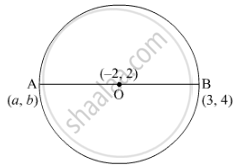# Find the Coordinates of Point A, Where Ab is a Diameter of the Circle with Centre (–2, 2) and B is the Point with Coordinates (3, 4). - Mathematics

Sum

Find the coordinates of point A, where AB is a diameter of the circle with centre (–2, 2) and B is the point with coordinates (3, 4).

#### SolutionLet the centre of the circle be O.
Since AB is the diameter so, O is the midpoint of AB.
Thus, using the section formula,

(a+3)/(2) = - 2

⇒ a = -4 - 3 = -7

And

(b + 4)/(2) = 2

⇒ b = 4 - 4 = 0

So, the coordinate of point A is (-7,0).

Is there an error in this question or solution?
2018-2019 (March) Delhi Set 2

Share